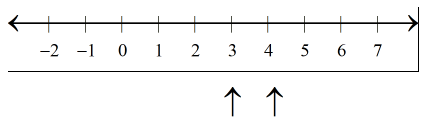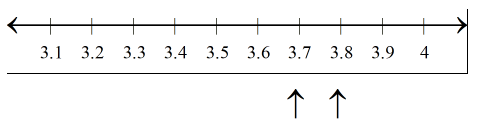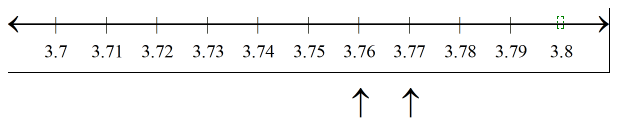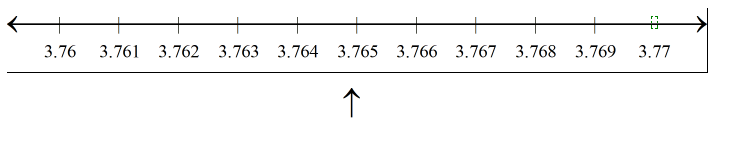Courses
Courses for Kids
Free study material
Offline Centres
MoreLast updated date: 03rd Dec 2023
Total views: 384.9k
Views today: 4.84k

# Visualise 3.765 on the number line, using successive magnification.Verified
384.9k+ views
Hint- In such a type of question, we start by successive magnification, and successively decrease the lengths of the portions of the number line in which 3.765 is present.

A number line is a line representing infinites of number shown in below number line.As we know that number 3.765 lies between 3and 4. So our first job is to find the number 3 and 4 on the number line. And we have to divide the gap between 3 and 4 into ten parts to see the number till 1st decimal place.
Hence by dividing 3 and 4 into ten parts we got 3, 3.1, 3.2, 3.3, 3.4, 3.5 ,3.6, 3.7 ,3.8, 3.9 ,4 and on representing on number line-Here, if we see more precisely, we can observe that the number 3.765 lies between $3.7$ and 3.8.
Now on further dividing the gap between 3.7 and 3.8 into ten parts
We shall get 3.7,3.71,3.72,3.73,3.74,3.75,3.76,3.77,3.78,3.79,3.8. And on representing these numbers on number line-Now on further observing more closely, the number 3.765 lies between 3.76 and 3.77.
Divide the gap between 3.76 and 3.77 into ten parts.
So we get 3.761, 3.762, 3.763, 3.764, 3.765, 3.766, 3.767, 3.768, 3.769, 3.77.
Then finally we got 3.765.Therefore 3.765 is visualized on the number line as shown in the above number line.

Note-Whenever we face such a type of problem, find how many digits are there after decimal places, then that many times you have to divide the number line between successive digits.